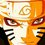# Synthetic Geometry Group - Surya Prakash's Proposal

This is my submission to Xuming's Synthetic Geometry Group. These problems are taken from different Olympiads. Try them on your own. I will soon post the solutions. Feel free to post the solutions.

1. Let $\Gamma$ be the circumcircle of $\Delta ABC$, and let $l$ be the tangent of $\Gamma$ passing through $A$. Let $D$, $E$ be the points on side $AB$ and $AC$ such that $BD :DA = AE : EC$. Line $DE$ meets $\Gamma$ at points $F$, $G$. The line parallel to $AC$ passing $D$ meets $l$ at $H$, the line parallel to $AB$ passing $E$ meets $l$ at $I$. Prove that $F$, $G$, $H$, $I$ are cyclic and BC is tangent to the circle through these points.

2. In $\Delta ABC$, let $H$ be the orthocenter of the triangle and $M$ be the midpoint of the side $BC$. Let the line perpendicular to $HM$ through $H$ meet $AC$ and $AB$ at $E$ and $F$. Prove that $HE = HF$. (Proposed by Xuming).

3. Let $\Delta ABC$ be an acute triangle with $D$, $E$, $F$ the feet of the altitudes lying on $BC$, $CA$, $AB$ respectively. One of the intersection points of the line $EF$ and the circumcircle is $P$. The lines $BP$ and $DF$ meet at point $Q$. Prove that AP= AQ.

4. Let $P$ be a point inside triangle $\Delta ABC$. Lines $AP$, $BP$, $CP$ meet the circumcircle of $\Delta ABC$ again at points $K$, $L$, $M$ respectively. The tangent to the circumcircle at $C$ meets line $AB$ at $S$. Prove that MK=ML if and only if $SP=SC$.Note by Surya Prakash
5 years, 10 months ago

This discussion board is a place to discuss our Daily Challenges and the math and science related to those challenges. Explanations are more than just a solution — they should explain the steps and thinking strategies that you used to obtain the solution. Comments should further the discussion of math and science.

When posting on Brilliant:

• Use the emojis to react to an explanation, whether you're congratulating a job well done , or just really confused .
• Ask specific questions about the challenge or the steps in somebody's explanation. Well-posed questions can add a lot to the discussion, but posting "I don't understand!" doesn't help anyone.
• Try to contribute something new to the discussion, whether it is an extension, generalization or other idea related to the challenge.

MarkdownAppears as
*italics* or _italics_ italics
**bold** or __bold__ bold
- bulleted- list
• bulleted
• list
1. numbered2. list
1. numbered
2. list
Note: you must add a full line of space before and after lists for them to show up correctly
paragraph 1paragraph 2

paragraph 1

paragraph 2

[example link](https://brilliant.org)example link
> This is a quote
This is a quote
    # I indented these lines
# 4 spaces, and now they show
# up as a code block.

print "hello world"
# I indented these lines
# 4 spaces, and now they show
# up as a code block.

print "hello world"
MathAppears as
Remember to wrap math in $$ ... $$ or $ ... $ to ensure proper formatting.
2 \times 3 $2 \times 3$
2^{34} $2^{34}$
a_{i-1} $a_{i-1}$
\frac{2}{3} $\frac{2}{3}$
\sqrt{2} $\sqrt{2}$
\sum_{i=1}^3 $\sum_{i=1}^3$
\sin \theta $\sin \theta$
\boxed{123} $\boxed{123}$

Sort by:

- 5 years, 10 months ago

This proposal*

Will check it out :)

- 5 years, 10 months ago

Nice problems!

- 5 years, 10 months ago

Nice ones man!

- 5 years, 10 months ago

Nicce problems! I just want to point out that I did not propose that problem. It can be viewed as a simple application of the Butterfly theorem(do you see it?). A "pesudo" generalization of this was utilized in one of my recent problems though.

- 5 years, 10 months ago

no problem. by the way I got the solution for that.

- 5 years, 10 months ago

is the condition if and only if in question 4 right?

- 5 years, 6 months ago